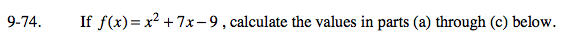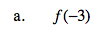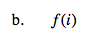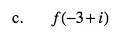### Home > A2C > Chapter 9 > Lesson 9.2.1 > Problem9-74

9-74.
1. If f (x) = x2 + 7x − 9, calculate the values in parts (a) through (c) below. Homework Help ✎

1. f (−3)

2. f (i)

3. f (−3 + i)−21f(i) = i 2 + 7i − 9

−10 + 7if(−3 + i) = (−3 +i)2 + 7(−3 + i) − 9